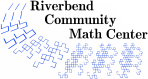# Do You Want to Know a Secret?

### Description

Students explore function rules and linear relationships through input and output tables and tile patterns.

30 minutes

5 to 8 lessons

### Topics

• patterns
• input/output tables
• functions
• variable expressions
• dependent and independent variables
• ordered pairs
• graphing linear equations

### Goals

• Students will be able to describe a pattern using words.
• Students will be able to describe a pattern using variables.
• Students will be able to create multiple representations of a linear function: equation, table, and graph.
• Students will be introduced to rate of change and slope.

### Materials

• square tiles (approximately 20 per student)
• large chart paper to display in the room
• grid paper
• Cartesian plane sheets
• rulers
• standard calculators
• student activity sheets - "Function Game" - "Secret Calculators" - "Tile Patterns Activity 1" - "Tile Patterns Activity 2"
• 3'' x 5'' cards
• TI-73 graphing calculators (for extension)

### Prerequisites

• Students are able to write and evaluate variable expressions.
• Students are able to graph coordinates in the Cartesian plane.

### Credits

• 1. West, Kelsch, Sowers; ''Algebraic thinking: Becoming Proficient with Patterns'', NCTM Conference, Indianapolis, April, 2011
• 2. Rubenstein, ''The Function Game'', Mathematics Teaching in the Middle School, Nov.-Dec. 1996.
• 3. Van de Walle and Lovin, Teaching Student Centered Mathematics Grades 5-8, Vol. 3, Pearson, 2009.

### Authors

Cheryl A. Nix, M.S.

### National Common Core Standards

 5.OA.1 Use parentheses, brackets, or braces in numerical expressions and evaluate expressions with these symbols. 5.OA.2 Write simple expressions that record calculations with numbers, and interpret numerical expressions without evaluating them. 5.OA.3 Generate a number or shape pattern that follows a given rule. Identify apparent features of the pattern that were not explicit in the rule itself. (For example, notice that when generating numbers by successively adding three that evens alternate with odds, and reason about why.) 6.EE.2 Write, read, and evaluate expressions in which letters stand for numbers. 6.EE.4 Identify when two expressions are equivalent and understand what equivalence of expressions implies. 6.EE.5 Understand solving an equation or inequality as a process for finding values that make a number sentence true. 6.EE.6 Use variables to represent numbers and write expressions when solving real-world or mathematical problems. 7.EE.1 Apply properties of operations as strategies to add, subtract, factor, and expand linear expressions with rational coefficients. 7.EE.2 Understand that rewriting an expression in different forms in a problem context can shed light on the problem and how the quantities in it are related. 7.EE.3 Solve multi-step real-life and mathematical problems posed with positive and negative rational numbers, using tools strategically. 8.EE.5 Graph proportional relationships, interpreting the unit rate as the slope of the graph. Compare two different proportional relationships represented in different ways. 8.F.1 Understand that a function is a rule that assigns to each input exactly one output, and that a graph of a function is the set of corresponding ordered pairs. 8.F.3 Interpret the equation y=mx+b as defining a linear function, whose graph is a straight line; give examples of functions that are not linear. 8.F.4 Construct a function to model a linear relationship between two quantities. 8.F.5 Describe qualitatively the functional relationship between two quantities by analyzing a graph (e.g. where the function is increasing or decreasing, linear or nonlinear).
Riverbend Community Math Center
hello@riverbendmath.org
http://riverbendmath.org
(574) 339-9111This work placed into the public domain by the Riverbend Community Math Center.